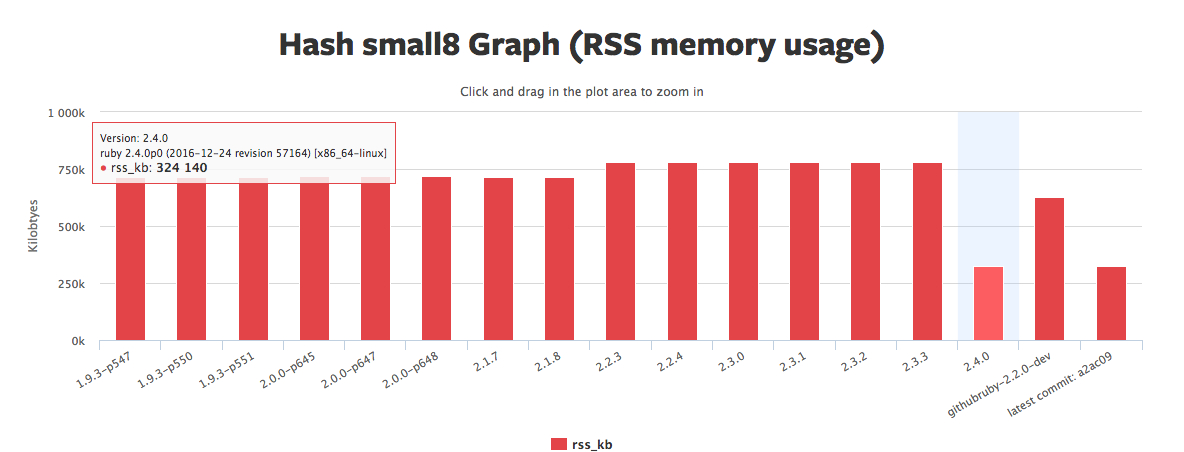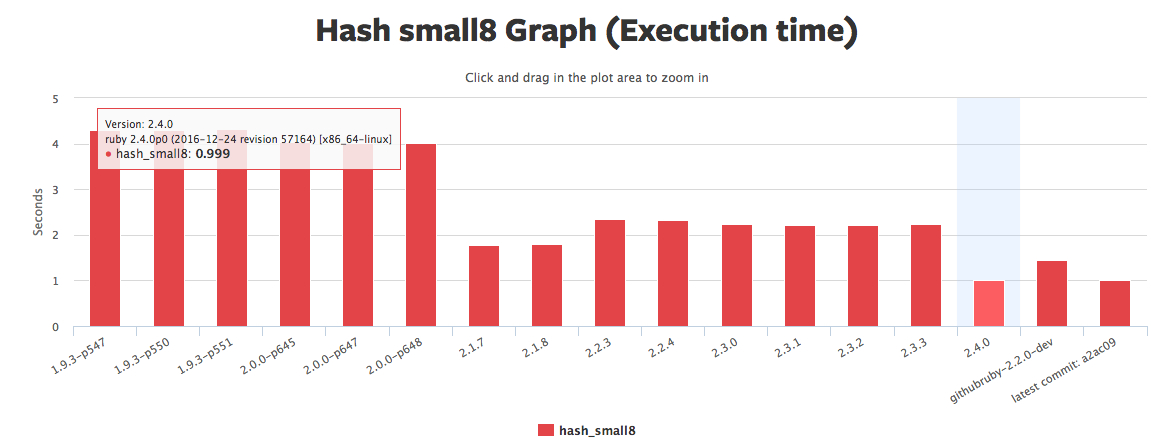# Ruby Ruby 2.4 哈希表 (Hash) 的变化

quakewang · 2017年03月16日 · 最后由 ji.menbi 回复于 2017年05月31日 · 7587 次阅读

Ruby 2.4 对哈希表引入了一些性能改进，这些变化是 Vladimir Makarov 在 2016 年初向 Ruby Hash 提交补丁时提出的

### Ruby 是如何实现哈希表的

``````class TurboHash

def initialize
@table = []
end
end
``````

``````class TurboHash
def [](key)
find(key).last
end

def find(key)
# 遍历数组查找对应的条目
@table.find do |entry|
key == entry.first
end
end

def []=(key, value)
if entry = find(key)
entry = value
else
@table << [key, value]
end
end
end
``````

``````require "benchmark"

legacy = Hash.new
turbo  = TurboHash.new

n = 10_000

def set_and_find(target)
key = rand
target[key] = rand
target[key]
end

Benchmark.bm do |x|
x.report("Hash: ") { n.times { set_and_find(legacy) } }
x.report("TurboHash: ") { n.times { set_and_find(turbo) } }
end
``````

``````       user     system      total        real
Hash:   0.010000   0.000000   0.010000 (  0.004094)
TurboHash:  10.070000   0.050000  10.120000 ( 10.240642)
````````````class Node

def initialize(o, n)
@object = o
@next = n
end
end

class TurboHash
NUM_BINS = 11

def initialize
@table = Array.new(NUM_BINS)
end

def [](key)
if node = node_for(key)
begin
return node.object if node.object == key
end while node = node.next
end
end

def node_for(key)
@table[index_of(key)]
end

def index_of(key)
key.hash % NUM_BINS
end

def []=(key, value)
@table[index_of(key)] = Node.new([key, value], node_for(key))
end
end
``````

``````       user     system      total        real
Hash:   0.010000   0.000000   0.010000 (  0.003859)
TurboHash:   0.010000   0.000000   0.010000 (  0.014998)
``````

``````def set_and_find(target)
keys = Array.new(30000) {rand}
keys.each do |key|
target[key] = rand
end.shuffle.each do |key|
target[key]
end
end
``````

``````       user     system      total        real
Hash:   0.020000   0.000000   0.020000 (  0.019680)
TurboHash:   2.360000   0.010000   2.370000 (  2.375549)
``````

``````class TurboHash
STARTING_BINS = 16

def initialize
@max_density = 5
@entry_count = 0
@bin_count   = STARTING_BINS
@table = Array.new(@bin_count)
end

def grow
@bin_count = @bin_count << 1
new_table = Array.new(@bin_count)
@table.each do |node|
if node
begin
new_index = index_of(node.object)
new_table[new_index] = Node.new(node.object, new_table[new_index])
end while node = node.next
end
end
@table = new_table
end

def full?
@entry_count > @max_density * @bin_count
end

def [](key)
if node = node_for(key)
begin
return node.object if node.object == key
end while node = node.next
end
end

def node_for(key)
@table[index_of(key)]
end

def index_of(key)
key.hash % @bin_count
end

def []=(key, value)
grow if full?
@table[index_of(key)] = Node.new([key, value], node_for(key))
@entry_count += 1
end
end
``````

``````       user     system      total        real
Hash:   0.020000   0.000000   0.020000 (  0.021225)
TurboHash:   0.090000   0.000000   0.090000 (  0.088481)
``````

### Ruby 2.4 之前的 2 个小细节优化

2.0 时候针对小于 7 个元素的 Hash 不额外创建链表结构，直接放置入数组，用数组遍历来实现，我们可以从性能测试代码中看到，达到 7 个元素的时候，性能有个阶梯式的差异：

``````require 'benchmark/ips'
Benchmark.ips do |x|
3.upto(10) do |i|
x.report(i) do
h = {}
i.times do |j|
h[j.to_s] = j
end
end
end
end
``````

2.2 时候，得益于 Object#hash 方法的改进，hash 值更加均匀分布，从质数取模改成了用位操作：

``````def index_of(key)
key.hash & @bin_count - 1
end
``````

### Ruby 2.4 Hash 的改变

Ruby 2.4 采用了Open addressing改进 Hash 的性能，和之前的实现方法差异主要在于：不再额外维护一个链表结构，每次都是按 hash 索引在数组插入，如果要插入的位置已经有元素存在，那么就找到一个空的位置插入。Ruby 2.4 采用了随机探测 ( Random Probing ) 来解决这个问题，最简单随机探测实现方法就是加一个伪随机值来探测下一个空位，伪随机值的作用是保证读取的时候随机值和放置时候一样，不会有性能问题。

``````irb> r = Random.new(7)
irb> r.rand(1..100)
# => 48
irb> r.rand(1..100)
# => 69
irb> r.rand(1..100)
# => 26

irb> r = Random.new(7)
irb> r.rand(1..100)
# => 48
irb> r.rand(1..100)
# => 69
irb> r.rand(1..100)
# => 26
``````

Ruby 2.4 采用了线性同余方法来实现一个伪随机值队列：

``````Xn+1 = (a * Xn + c ) % m
``````

1. m > 0
2. 0 < a < m
3. 0 <= c < m
4. 0 <= X0 < m

``````irb> a, x_n, c, m = [3, 1, 4, 16]

irb> x_n = (a * x_n + c) % m
=> 7
irb> x_n = (a * x_n + c) % m
=> 9
irb> x_n = (a * x_n + c) % m
=> 15
irb> x_n = (a * x_n + c) % m
=> 1
``````

1. m 和 c 互质
2. (a - 1) 能被 m 的所有质因数整除
3. 如果 m 能被 4 整除，那么 (a - 1) 也必须能被 4 整除

``````irb> a, x_n, c, m = [5, 7, 1, 16]

irb> x_n = (a * x_n + c) % m
# => 4
irb> x_n = (a * x_n + c) % m
# => 5
irb> x_n = (a * x_n + c) % m
# => 10

irb> (1..16).map { x_n = (a * x_n + c) % m }.sort
# => [0, 1, 2, 3, 4, 5, 6, 7, 8, 9, 10, 11, 12, 13, 14, 15]
``````https://blog.heroku.com/ruby-2-4-features-hashes-integers-rounding#hash-changes

http://blog.redpanthers.co/behind-scenes-hash-table-performance-ruby-2-4/

Open addressing 可以减少 hash 碰撞情况下的内存占用 (不需要海量内存来存指针啦), 少了指针跳转减少 CPU cache miss

Open addressing 探测下一个可用 slot 的方式叫 probing.

Pseudo random probing 相比最简单的 linear probing, 可以减少 worst case probing 的遍历 (这种情况叫 primary clustering), 但是也有可能会增加一丢丢 cache miss ...

(不需要海量内存来存指针啦) 对于这句话不是很理解，能麻烦深入讲解一下么， 感谢